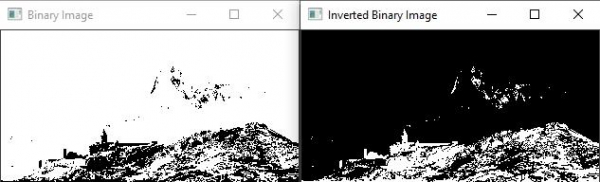# How to invert a binary image in OpenCV using C++?

Inverting a binary image means inverting the pixel values. From a visual perspective, when we invert a binary image, white pixels will be converted to black, and black pixels will be converted to white.

The basic form of this function is −

cvtColor(original_image, grayscale_image, COLOR_BGR2GRAY);

The next line is converting the grayscale image into a binary image and storing the converted image into 'binary_image' matrix.

threshold(grayscale_image, binary_image, 100, 255, THRESH_BINARY);

Here 'grayscale_image' is the source matrix, 'binary_image' is the destination matrix. After that, there are two values 100 and 255. These two values represent the threshold range. In this line, the threshold range represents the grayscale pixel values to be converted.

bitwise_not(source matrix, destination matrix);

The bitwise_not() function inverse the source matrix's pixel values and store them in the destination matrix. The source matrix is 'binary_image', and the destination matrix is 'inverted_binary_image'.

The following program performs the binary image inversion −

## Example

#include<iostream>
#include<opencv2/highgui/highgui.hpp>
#include<opencv2/imgproc/imgproc.hpp>
using namespace cv;
using namespace std;
int main(int argc, char** argv) {
Mat original_image;//declaring a matrix to load the original image//
Mat grayscale_image;//declaring a matrix to store converted image//
Mat binary_image;//declaring a matrix to store the binary image
Mat inverted_binary_image;//declaring a matrix to store inverted binary image
namedWindow("Binary Image");//declaring window to show binary image//
namedWindow("Inverted Binary Image");//declaring window to show inverted binary image//
cvtColor(original_image, grayscale_image,COLOR_BGR2GRAY);//Converting BGR to Grayscale image and storing it into 'converted' matrix//
threshold(grayscale_image, binary_image, 100, 255, THRESH_BINARY);//converting grayscale image stored in 'converted' matrix into binary image//
bitwise_not(binary_image, inverted_binary_image);//inverting the binary image and storing it in inverted_binary_image matrix//
imshow("Binary Image", binary_image);//showing binary image//
imshow("Inverted Binary Image", inverted_binary_image);//showing inverted binary image//
waitKey(0);
return 0;
}

## Output